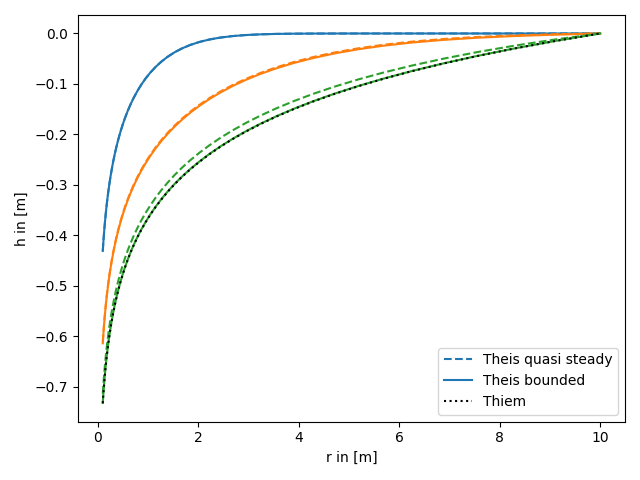The quasi steady is reached, when the radial shape of the drawdown in not changing anymore.```import numpy as np
from matplotlib import pyplot as plt

from anaflow import theis, thiem

time = [10, 100, 1000]
r_ref = 10.0

time,
storage=1e-3,
transmissivity=1e-4,
rate=-1e-4,
)
)
time, rad, storage=1e-3, transmissivity=1e-4, rate=-1e-4, r_bound=r_ref
)

for i, step in enumerate(time):
label_1 = "Theis quasi steady" if i == 0 else None
label_2 = "Theis bounded" if i == 0 else None